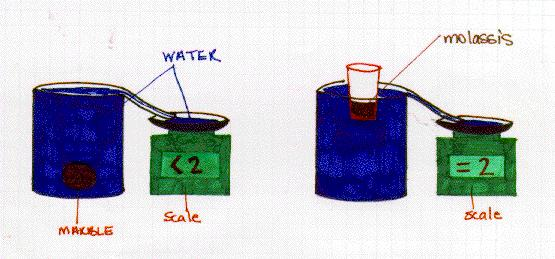Why Objects Float

We asked the students to predict what objects would float when thrown into an aquarium filled with water. We threw in keys, marbles, rubber corks and ping-pong balls. We then asked the students to explain why some objects float and others do not. The students had a hard time explaining why.

Why do objects float?

Any object dropped into a liquid displaces that liquid. A floating object weighs the same as the liquid it displaces. An object that sinks, weighs more than the liquid it displaces.To show this through a visual demonstration we dropped a marble weighing 2 grams into a beaker of water. The marble sank to the bottom. We asked the students to predict how much water would pour out onto the scale since the marble sank to the bottom. The answer was less than 2 grams.

We then took a styrofoam cup filled with molassis which weighed 2 grams in total and dropped it into the water. The cup floated on the water. We asked the students to tell us how much water should pour out onto the scale. The answer was 2 grams.

By pouring more molassis into the cup you can explain to the class the loading of a freight liner.

ToBuoyancy